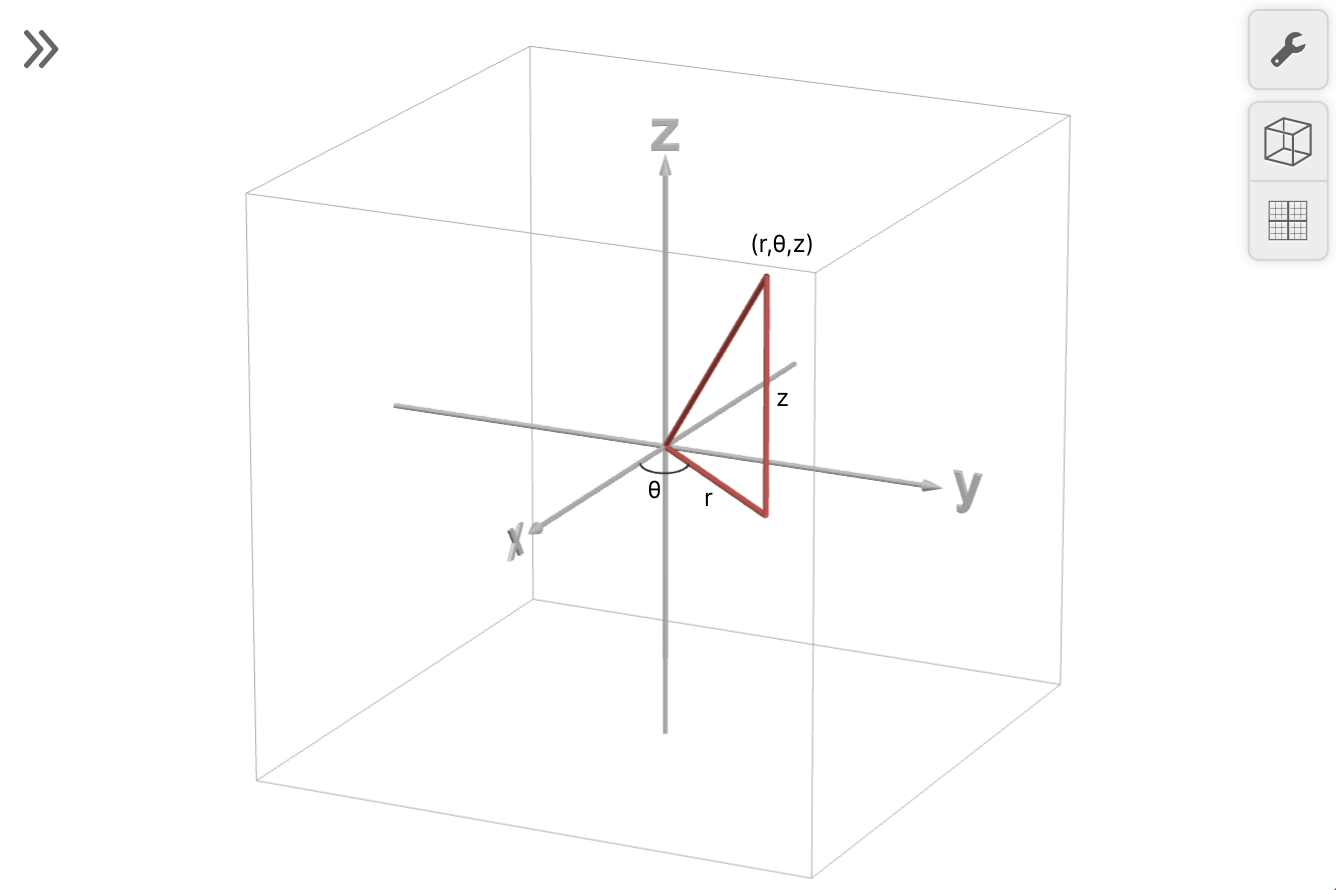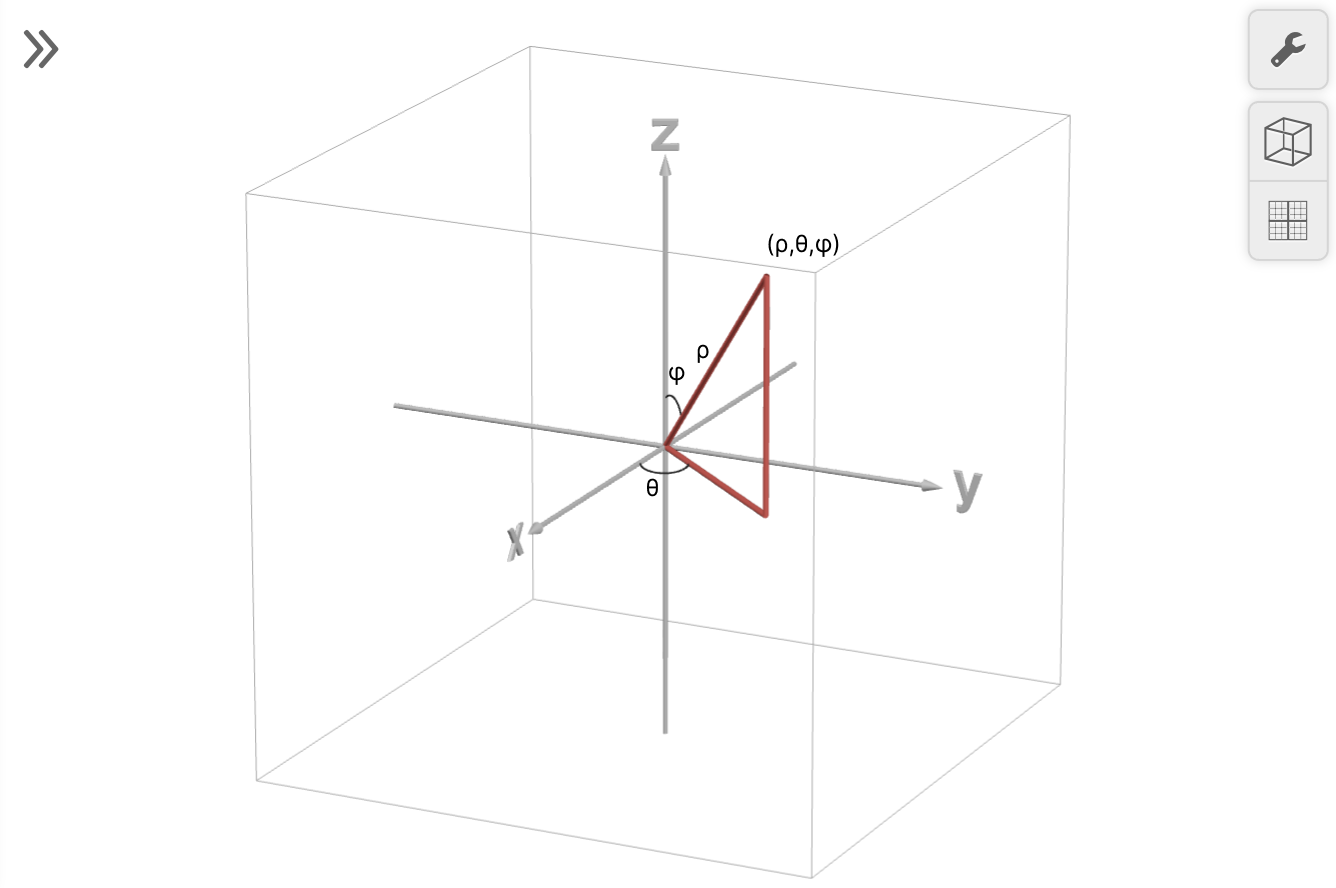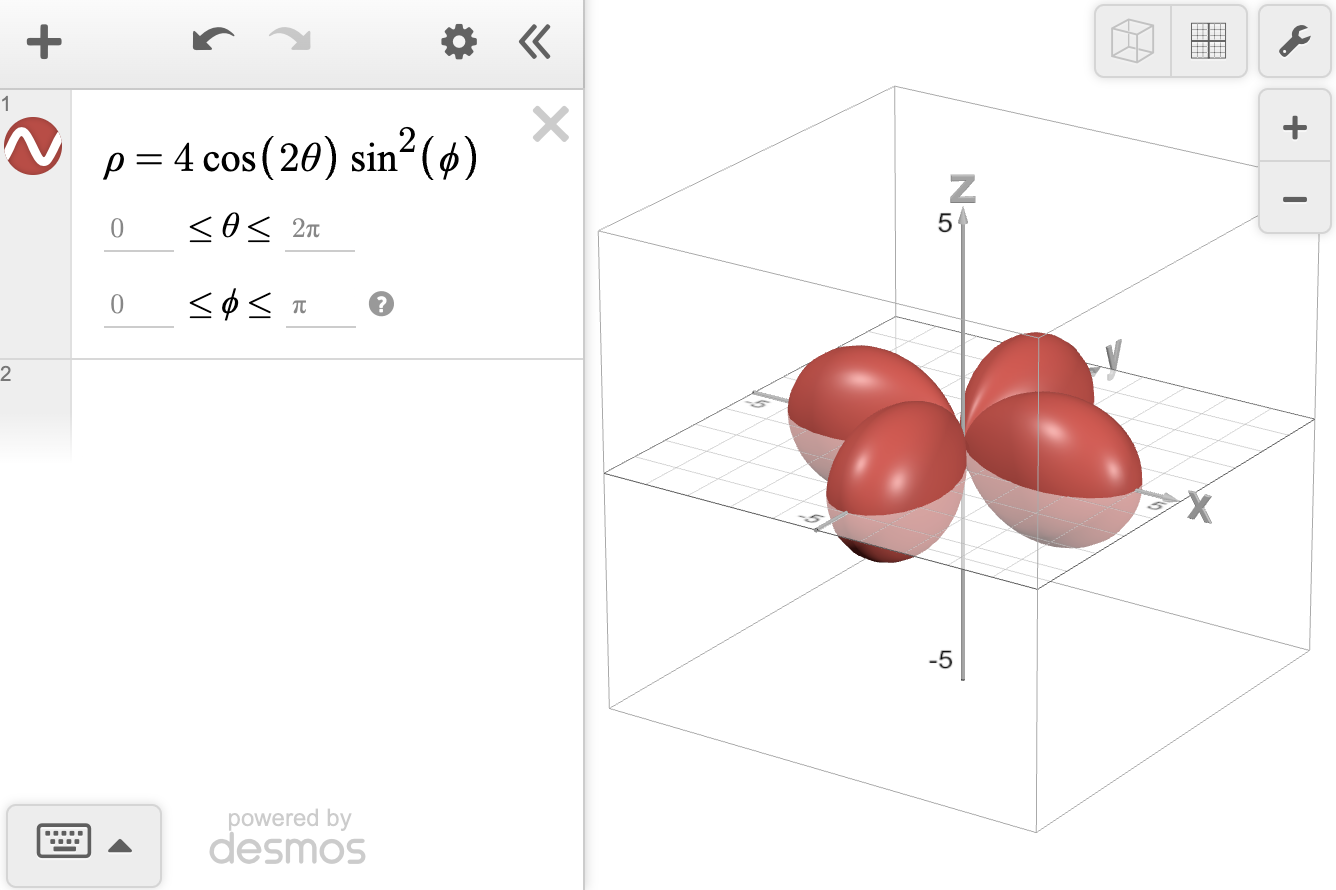# Cylindrical and Spherical Coordinates

### Non-Cartesian Systems

Cartesian coordinates can be used in both 2D and 3D. In many cases, however, it is more helpful to describe the location of a point using distance and direction.

For polar coordinates in 2D, $$r$$ is the distance from the origin to the point and theta ($$\theta$$) is the direction or the angle of rotation from the positive $$x$$-axis. We write the polar coordinates of a point as $$(r,\theta)$$ with $$\theta$$ usually given in radians. Although it doesn't plot polar points, the Desmos 2D graphing calculator considers any equation or inequality written in terms of $$r$$ and $$\theta$$ to be in polar form, and will plot these equations/inequalities as a polar curve or region. More information can be found in our Polar Graphing article.

### Cylindrical Coordinates

Extending this idea of polar coordinates to 3D gives us cylindrical coordinates. If we add a $$z$$ coordinate, then a cylindrical point looks like $$(r, θ, z)$$ and combines polar coordinates in the $$XY$$ plane with the Cartesian coordinate $$z$$ in 3D. Again, points in this form cannot be directly plotted in the Desmos calculator, but Desmos will recognize equations written in terms of $$r$$, $$θ$$, and $$z$$ to be cylindrical. For example, $$r = 2$$ will graph a circle with radius 2. To view the cylinder, check the 'Extend to 3D' checkbox as seen in this example graph.### Spherical Coordinates

Another way to describe the location of a point using distance and direction in 3D is with spherical coordinates. Spherical coordinates use rho ($$ρ$$) as the distance between the origin and the point, whereas for cylindrical points,  $$r$$ is the distance from the origin to the projection of the point onto the XY plane.  For spherical coordinates, instead of using the Cartesian $$z$$, we use phi ($$φ$$) as a second angle. A spherical point is in the form $$(ρ, θ, φ)$$ where $$φ$$ is the angle between the ray from the origin and the positive $$z$$-axis as shown. Try typing $$ρ = 2$$ to graph a sphere with radius 2 as seen in this example graph.It is important to note that not all disciplines use the same convention for spherical coordinates. For example, physics texts commonly use spherical coordinates in the form $$(ρ, θ, φ)$$ where $$θ$$ is the polar angle to the $$z$$-axis and $$φ$$ is the azimuthal angle (switched from what Desmos 3D is using).

### Graphing with Spherical Coordinates in Desmos

When you write an equation in the form $$ρ$$ equals a function of $$θ$$ and $$φ$$ in the Desmos 3D calculator, it will use spherical coordinates. For example, try plotting the magnitude of the real spherical harmonic function $$Y_2^2$$ in Desmos 3D.

To type the $$ρ$$ symbol, type the word 'rho.' Similarly for the $$θ$$ symbol, type the word ‘theta’ and for the $$φ$$ symbol, type ‘phi.’

The default domain is that $$θ$$ plots between $$0$$ and $$2\pi$$ and $$φ$$ plots between $$0$$ and $$\pi$$. You can change these bounds, but the defaults are set to best display unique spherical coordinates.